Next: Surface Integrals Up: Vectors and Vector Fields Previous: Line Integrals

# Vector Line Integrals

A vector field is defined as a set of vectors associated with each point in space. For instance, the velocity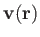in a moving liquid (e.g., a whirlpool) constitutes a vector field. By analogy, a scalar field is a set of scalars associated with each point in space. An example of a scalar field is the temperature distribution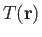in a furnace.

Consider a general vector field. Let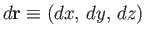be the vector element of line length. Vector line integrals often arise as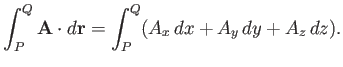(A.81)

For instance, if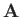is a force-field then the line integral is the work done in going from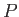to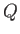.

For example, consider the work done by a repulsive inverse-square central field,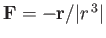. The element of work done is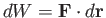. Take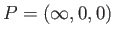and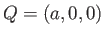. The first route considered is along the-axis, so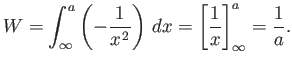(A.82)

The second route is, firstly, around a large circle (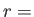constant) to the point (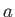,, 0), and then parallel to the-axis. (See Figure A.16). In the first part, no work is done, becauseis perpendicular to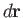. In the second part,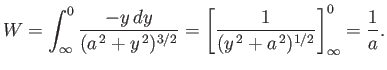(A.83)

In this case, the integral is independent of the path. However, not all vector line integrals are path independent.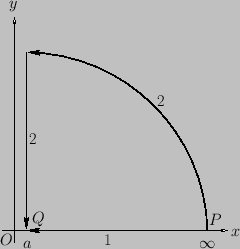Next: Surface Integrals Up: Vectors and Vector Fields Previous: Line Integrals
Richard Fitzpatrick 2016-03-31